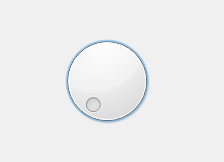# PyQt5 – QDial

QDial is a class in PyQt5 which provide user to select the value within a program-definable range, and the range either wraps around (for example, with angles measured from 0 to 359 degrees). Also, it can be used to show the current value in a similar way of speedometer. Below is how the QDial looks likeExample :
A window having a QDial and user can change the value it and when user change the value the current value will get displayed in the label

Below is the implementation

 `# importing libraries ` `from` `PyQt5.QtWidgets ``import` `*`  `from` `PyQt5 ``import` `QtCore, QtGui ` `from` `PyQt5.QtGui ``import` `*`  `from` `PyQt5.QtCore ``import` `*`  `import` `sys ` ` `  ` `  `class` `Window(QMainWindow): ` ` `  `    ``def` `__init__(``self``): ` `        ``super``().__init__() ` ` `  `        ``# setting title ` `        ``self``.setWindowTitle(``"Python "``) ` ` `  `        ``# setting geometry ` `        ``self``.setGeometry(``100``, ``100``, ``500``, ``400``) ` ` `  `        ``# calling method ` `        ``self``.UiComponents() ` ` `  `        ``# showing all the widgets ` `        ``self``.show() ` ` `  `    ``# method for components ` `    ``def` `UiComponents(``self``): ` ` `  `        ``# creating QDial object ` `        ``dial ``=` `QDial(``self``) ` ` `  `        ``# setting geometry to the dial ` `        ``dial.setGeometry(``100``, ``100``, ``100``, ``100``) ` ` `  `        ``# adding action to the dial ` `        ``dial.valueChanged.connect(``lambda``: dial_method()) ` ` `  `        ``# creating a label ` `        ``label ``=` `QLabel(``"GeeksforGeeks"``, ``self``) ` ` `  `        ``# setting geometry to the label ` `        ``label.setGeometry(``220``, ``125``, ``200``, ``60``) ` ` `  `        ``# making label multiline ` `        ``label.setWordWrap(``True``) ` ` `  `        ``# method called by the dial ` `        ``def` `dial_method(): ` ` `  `            ``# getting dial value ` `            ``value ``=` `dial.value() ` ` `  `            ``# setting text to the label ` `            ``label.setText(``"Current Value : "` `+` `str``(value)) ` ` `  ` `  `# create pyqt5 app ` `App ``=` `QApplication(sys.argv) ` ` `  `# create the instance of our Window ` `window ``=` `Window() ` ` `  `# start the app ` `sys.exit(App.``exec``()) `

Output :

My Personal Notes arrow_drop_upCheck out this Author's contributed articles.

If you like GeeksforGeeks and would like to contribute, you can also write an article using contribute.geeksforgeeks.org or mail your article to contribute@geeksforgeeks.org. See your article appearing on the GeeksforGeeks main page and help other Geeks.

Please Improve this article if you find anything incorrect by clicking on the "Improve Article" button below.

Article Tags :

Be the First to upvote.

Please write to us at contribute@geeksforgeeks.org to report any issue with the above content.